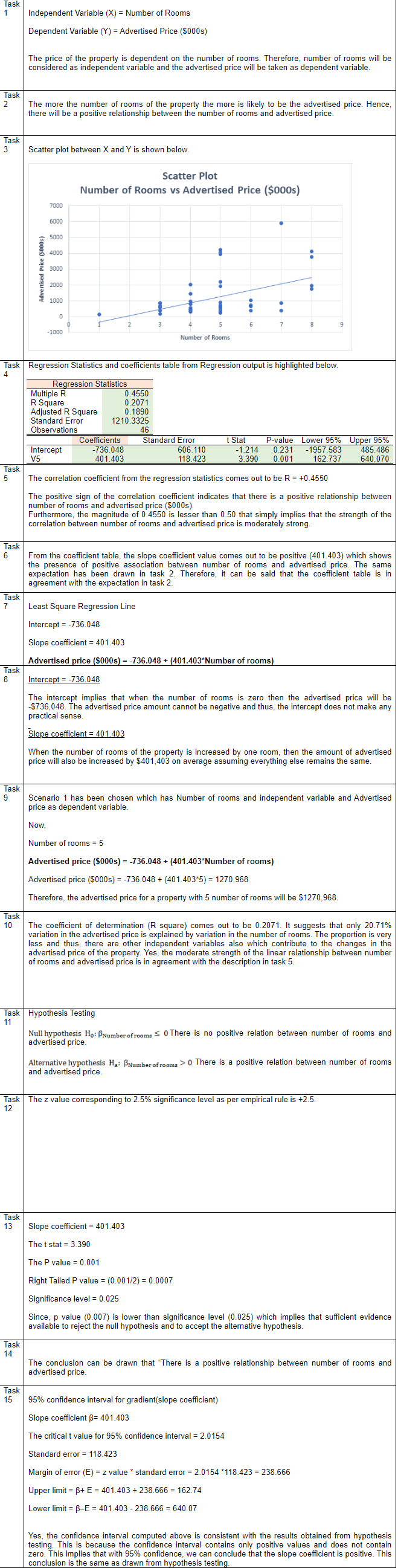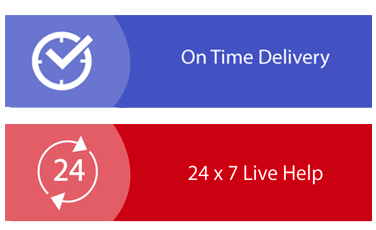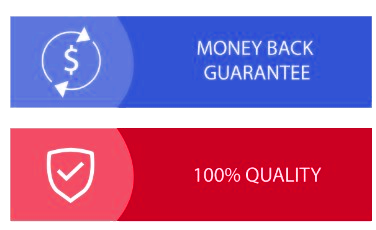× Limited Time Offer ! FLAT 30-40% off - Grab Deal Before It’s Gone. Order Now

## BEO1106 Business Statistics Assignment Sample

### Introduction

The price of a property can be determined by a number of factors (in addition to the market
trend). These factors may include (but not the least): The location, the land size, the size of the built area, the building type, the property type, number of rooms, number of bathroom and toilets, swimming pool, tennis court and so on.

The sample data you collected for your assignment contain the following variables:

V1 = Region where property is located (1 = North, 2 = West, 3 = East, 4 = Central)
V2 = Property type (0 = Unit, 1 = House)
V3 = Sale result (1 = Sold at auction, 2 = Passed-in, 3 = Private sale, 4 = Sold before auction).
Note that a blank cell for this variable indicates that the property did not sell.
V4 = Building type (1 = Brick, 2 = Brick veneer, 3 = Weatherboard, 4 = Vacant land)
V5 = Number of rooms
V6 = Land size (Square meters)
V7 = Sold Price (\$000s)

### Requirement

In relation to the Simple Regression topic of Business Statistics, for this Case Study, you are
required to conduct a regression analysis to estimate the relation between Number of Rooms and Advertised Price of properties in Melbourne.

### Instruction

You need to prepare a sample data using the Number of Rooms and the Advertised Price variables. You may find that V5 (Number of Rooms) variable has some missing observations in your sample. In order for Excel to estimate a regression equation, Excel requires a balanced data set. This means that both dependent variables and independent variables must have the same (balanced) number of observations in the data set. To balance the data set, we have to remove the observations which contain missing data. Refer to the steps in the Excel file Regression Estimation example for Case Study.xlsx to assist you to construct your balanced sample data set for the regression analysis.

In the Answer Sheet provided, name the dependent variable (Y) and the independent variable (X). Provide a brief explanation for assignment help to support your choice.

In a sentence, explain whether you expect a positive or a negative relation between the X and the Y variables.

Use Excel to produce a scatterplot using the independent variable for the horizontal (X) axis and the dependent variable as the vertical (Y) axis. Copy and paste the scatterplot to the Answer Booklet.

Hint: Follow the graph presentation (in Step 5, Regression Estimation example for Case
Study.xlsx).

Note:Title of the scatterplot and the labels for axes will account for 0.5 mark for each.

Follow the Excel procedure (select Data / Data Analysis / Regression) outlined on seminar note Slide 16, using the X variable and the Y variable you nominated in Task 1, generate regression estimation output tables. Copy the Regression Statistics and Coefficients tables (refer to Slide 27 and Slide 28) to the Answer Booklet.

Refer to the Regression Statistics table in Task 4, briefly describe the strength of the correlation between X and Y variables. Ensure your statement is supported by the statistic figure from the table.

Does the information shown in the Coefficients table agree with your expectation in Task 2?

Refer to the Coefficients table, and follow the presentation on seminar note Slide 19, construct the least squares linear regression equation for the relationship between the independent variable and the dependent variable.

Interpret the estimated intercept and the slope coefficients.

Select one of the two following scenarios which describe your choice in Task 1.

• In Task 1, if you nominated Number of Rooms is the independent variable, then you are asked to estimate the Advertised Price (dependent variable) of a property given the number of rooms of the property is 5.

• In Task 1, if you nominated Advertised Price is the independent variable, then you are asked to estimate the Number of Rooms (dependent variable) of a property given the advertised price is \$1.55 (million).

With reference to the R Square value provided in the Regression Statistics table, explain whether you would trust your estimation in Task 9. Comment on whether your answer in Task10 agrees with the answer in Task 5 in terms of the strength of the linear relationship between X and Y.

State, symbolically, the null and alternative hypotheses for testing whether there is a positive linear relationship between Number of Rooms and Advertised Price in the population.

Use the Empirical Rule, state the z- value which is corresponding to 2.5% significant level.

Use the p-value approach to decide, at a 2.5% level of significance, whether the null hypothesis of the test referred to in Task 11 can be rejected (or not). Make sure you provide a justification for your decision.

Following the decision in Task13, provide a precise conclusion to the hypothesis test conducted in Task 13.

From information provided in the Coefficients table, construct a 95% confidence interval estimate of the gradient of the population regression line. Is this interval consistent with the conclusion to the hypothesis test you arrived at in Task 14? Briefly explain the reasoning behind your answer

## SolutionFill the form to continue reading